# cs702 ppt.ppt

28. May 2023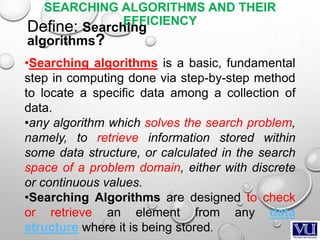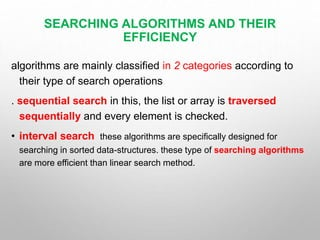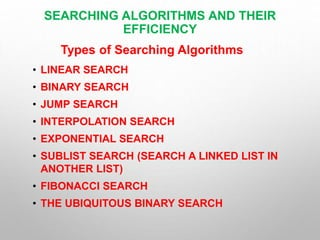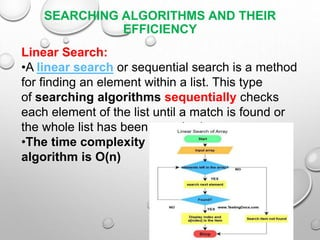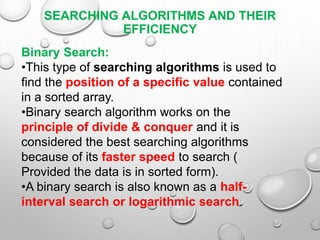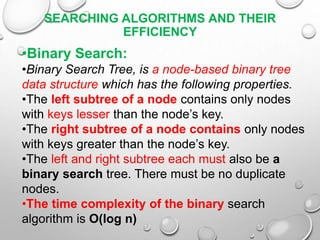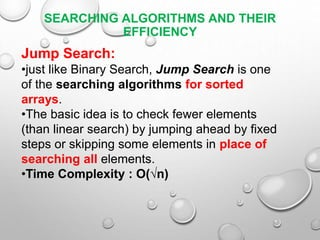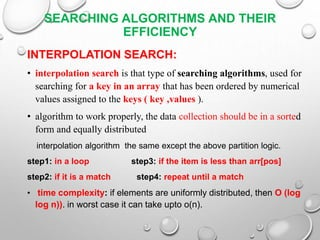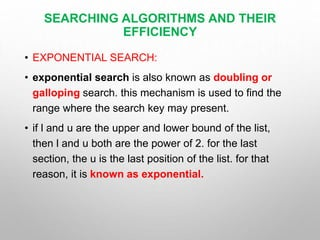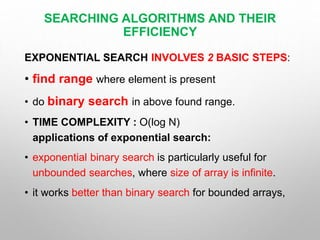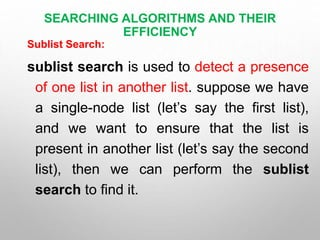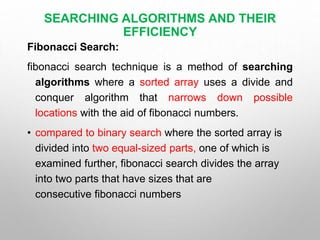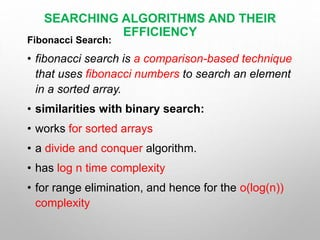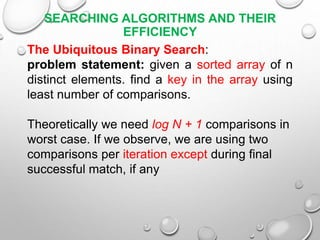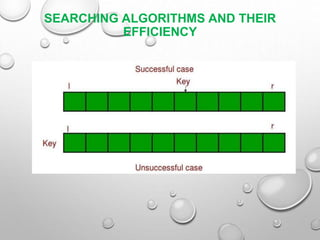1 von 19

### cs702 ppt.ppt

• 2. CS702 ADVANCED ALGORITHMS ANALYSIS AND DESIGN MUHAMMAD JIBREEL SAMMAR ID:(MS210400019)
• 3. MY TOPIC SEARCHING ALGORITHMS AND THEIR EFFICIENCY, CONSIDERING TIME COMPLEXITY
• 4. SEARCHING ALGORITHMS AND THEIR EFFICIENCY •Searching algorithms is a basic, fundamental step in computing done via step-by-step method to locate a specific data among a collection of data. •any algorithm which solves the search problem, namely, to retrieve information stored within some data structure, or calculated in the search space of a problem domain, either with discrete or continuous values. •Searching Algorithms are designed to check or retrieve an element from any data structure where it is being stored. Define: Searching algorithms?
• 5. algorithms are mainly classified in 2 categories according to their type of search operations . sequential search in this, the list or array is traversed sequentially and every element is checked. • interval search these algorithms are specifically designed for searching in sorted data-structures. these type of searching algorithms are more efficient than linear search method. SEARCHING ALGORITHMS AND THEIR EFFICIENCY
• 6. • LINEAR SEARCH • BINARY SEARCH • JUMP SEARCH • INTERPOLATION SEARCH • EXPONENTIAL SEARCH • SUBLIST SEARCH (SEARCH A LINKED LIST IN ANOTHER LIST) • FIBONACCI SEARCH • THE UBIQUITOUS BINARY SEARCH SEARCHING ALGORITHMS AND THEIR EFFICIENCY Types of Searching Algorithms
• 7. Linear Search: •A linear search or sequential search is a method for finding an element within a list. This type of searching algorithms sequentially checks each element of the list until a match is found or the whole list has been searched. •The time complexity algorithm is O(n) SEARCHING ALGORITHMS AND THEIR EFFICIENCY
• 8. Binary Search: •This type of searching algorithms is used to find the position of a specific value contained in a sorted array. •Binary search algorithm works on the principle of divide & conquer and it is considered the best searching algorithms because of its faster speed to search ( Provided the data is in sorted form). •A binary search is also known as a half- interval search or logarithmic search. SEARCHING ALGORITHMS AND THEIR EFFICIENCY
• 9. •Binary Search: •Binary Search Tree, is a node-based binary tree data structure which has the following properties. •The left subtree of a node contains only nodes with keys lesser than the node’s key. •The right subtree of a node contains only nodes with keys greater than the node’s key. •The left and right subtree each must also be a binary search tree. There must be no duplicate nodes. •The time complexity of the binary search algorithm is O(log n) SEARCHING ALGORITHMS AND THEIR EFFICIENCY
• 10. Jump Search: •just like Binary Search, Jump Search is one of the searching algorithms for sorted arrays. •The basic idea is to check fewer elements (than linear search) by jumping ahead by fixed steps or skipping some elements in place of searching all elements. •Time Complexity : O(√n) SEARCHING ALGORITHMS AND THEIR EFFICIENCY
• 11. INTERPOLATION SEARCH: • interpolation search is that type of searching algorithms, used for searching for a key in an array that has been ordered by numerical values assigned to the keys ( key ,values ). • algorithm to work properly, the data collection should be in a sorted form and equally distributed interpolation algorithm the same except the above partition logic. step1: in a loop step3: if the item is less than arr[pos] step2: if it is a match step4: repeat until a match • time complexity: if elements are uniformly distributed, then O (log log n)). in worst case it can take upto o(n). SEARCHING ALGORITHMS AND THEIR EFFICIENCY
• 12. • EXPONENTIAL SEARCH: • exponential search is also known as doubling or galloping search. this mechanism is used to find the range where the search key may present. • if l and u are the upper and lower bound of the list, then l and u both are the power of 2. for the last section, the u is the last position of the list. for that reason, it is known as exponential. SEARCHING ALGORITHMS AND THEIR EFFICIENCY
• 13. EXPONENTIAL SEARCH INVOLVES 2 BASIC STEPS: • find range where element is present • do binary search in above found range. • TIME COMPLEXITY : O(log N) applications of exponential search: • exponential binary search is particularly useful for unbounded searches, where size of array is infinite. • it works better than binary search for bounded arrays, SEARCHING ALGORITHMS AND THEIR EFFICIENCY
• 14. Sublist Search: sublist search is used to detect a presence of one list in another list. suppose we have a single-node list (let’s say the first list), and we want to ensure that the list is present in another list (let’s say the second list), then we can perform the sublist search to find it. SEARCHING ALGORITHMS AND THEIR EFFICIENCY
• 15. Fibonacci Search: fibonacci search technique is a method of searching algorithms where a sorted array uses a divide and conquer algorithm that narrows down possible locations with the aid of fibonacci numbers. • compared to binary search where the sorted array is divided into two equal-sized parts, one of which is examined further, fibonacci search divides the array into two parts that have sizes that are consecutive fibonacci numbers SEARCHING ALGORITHMS AND THEIR EFFICIENCY
• 16. Fibonacci Search: • fibonacci search is a comparison-based technique that uses fibonacci numbers to search an element in a sorted array. • similarities with binary search: • works for sorted arrays • a divide and conquer algorithm. • has log n time complexity • for range elimination, and hence for the o(log(n)) complexity SEARCHING ALGORITHMS AND THEIR EFFICIENCY
• 17. The Ubiquitous Binary Search: problem statement: given a sorted array of n distinct elements. find a key in the array using least number of comparisons. Theoretically we need log N + 1 comparisons in worst case. If we observe, we are using two comparisons per iteration except during final successful match, if any SEARCHING ALGORITHMS AND THEIR EFFICIENCY
• 18. SEARCHING ALGORITHMS AND THEIR EFFICIENCY
• 19. THANKS